# ASSURE MODEL LESSON PLAN IN MATHEMATICS 10 Lesson

• Slides: 51ASSURE MODEL: LESSON PLAN IN MATHEMATICS 10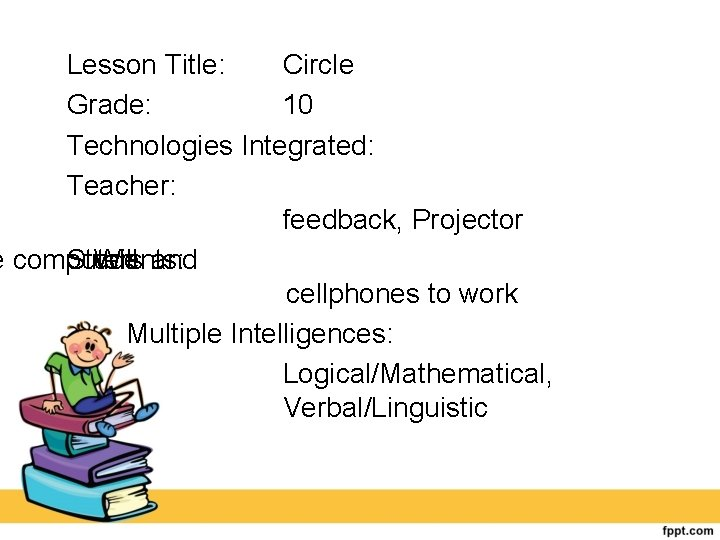Lesson Title: Circle Grade: 10 Technologies Integrated: Teacher: feedback, Projector e computers and Will Students: cellphones to work Multiple Intelligences: Logical/Mathematical, Verbal/Linguistic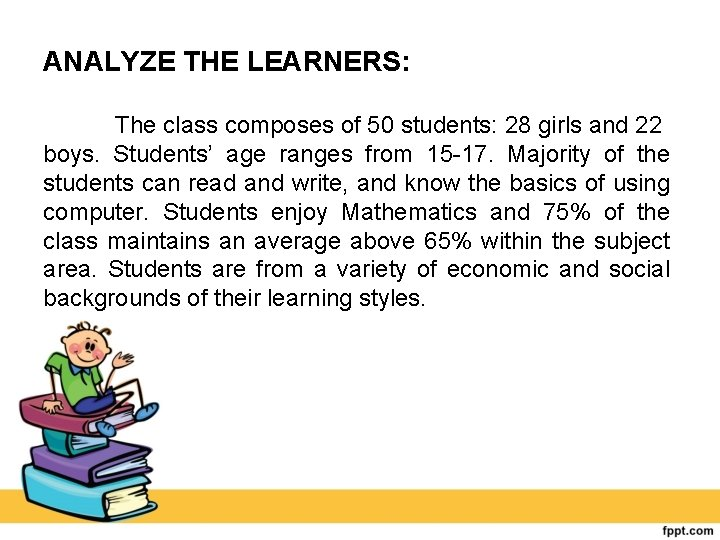ANALYZE THE LEARNERS: The class composes of 50 students: 28 girls and 22 boys. Students’ age ranges from 15 17. Majority of the students can read and write, and know the basics of using computer. Students enjoy Mathematics and 75% of the class maintains an average above 65% within the subject area. Students are from a variety of economic and social backgrounds of their learning styles.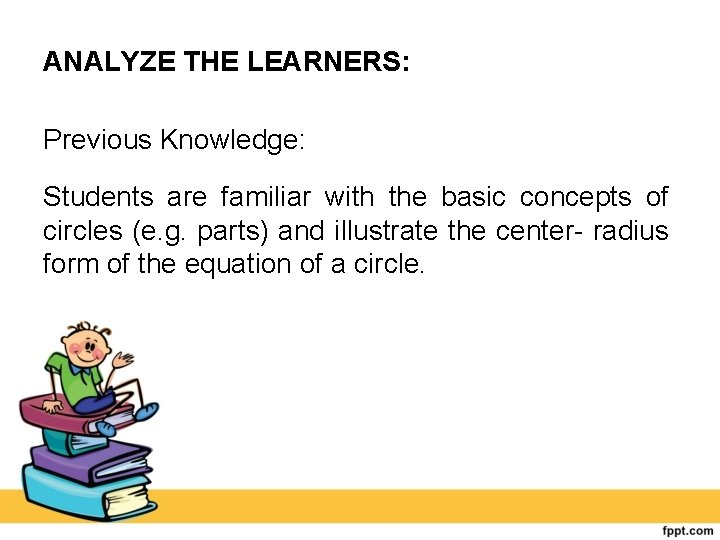ANALYZE THE LEARNERS: Previous Knowledge: Students are familiar with the basic concepts of circles (e. g. parts) and illustrate the center radius form of the equation of a circle.OBJECTIVES Within the period, students are expected to: Content Standard: a. Demonstrates understanding of key concepts of circle and coordinate geometry.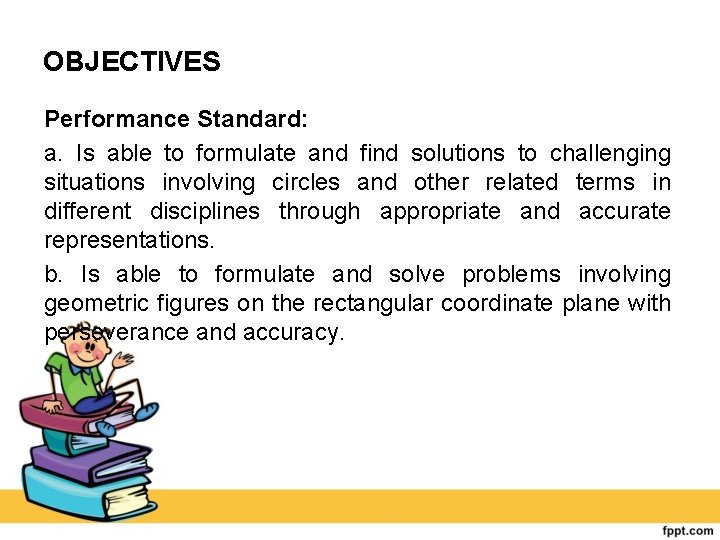OBJECTIVES Performance Standard: a. Is able to formulate and find solutions to challenging situations involving circles and other related terms in different disciplines through appropriate and accurate representations. b. Is able to formulate and solve problems involving geometric figures on the rectangular coordinate plane with perseverance and accuracy.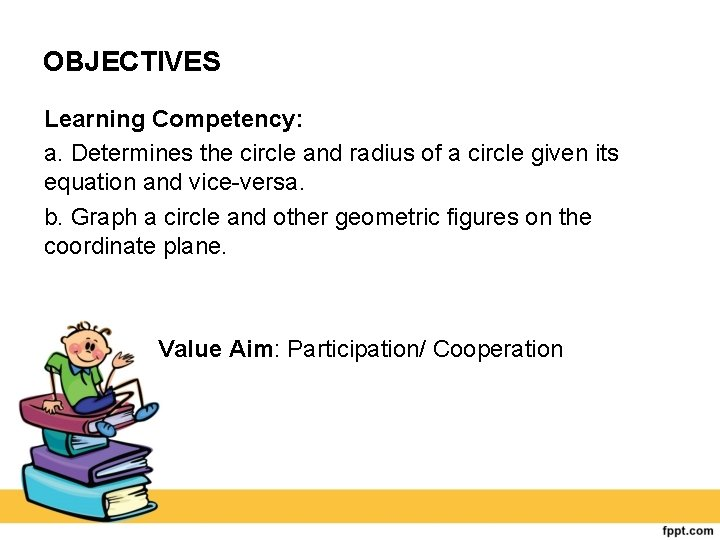OBJECTIVES Learning Competency: a. Determines the circle and radius of a circle given its equation and vice versa. b. Graph a circle and other geometric figures on the coordinate plane. Value Aim: Participation/ CooperationSELECT AND UTILIZE TEACHING METHODS/STRATEGIES: Discussion, question and answer along with the technological tools will be used to teach students how illustrate, determine and graph a circle. SELECT AND UTILIZE MEDIA: a) b) c) d) Laptop Projector LCD screen Geo. Gebra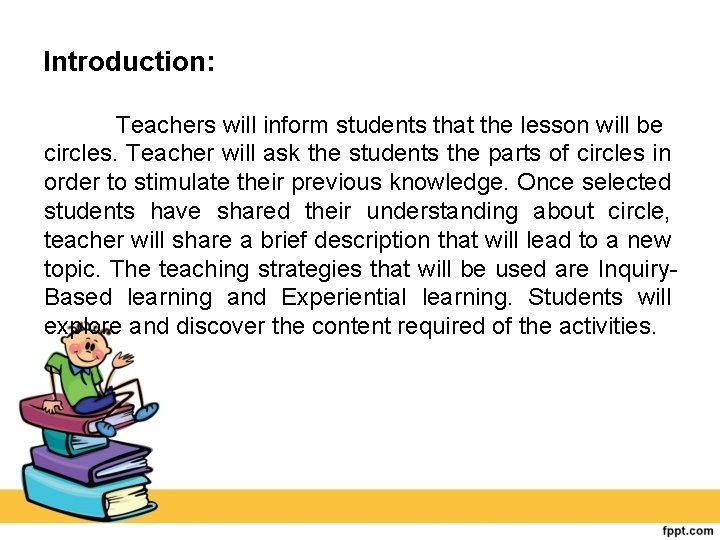Introduction: Teachers will inform students that the lesson will be circles. Teacher will ask the students the parts of circles in order to stimulate their previous knowledge. Once selected students have shared their understanding about circle, teacher will share a brief description that will lead to a new topic. The teaching strategies that will be used are Inquiry Based learning and Experiential learning. Students will explore and discover the content required of the activities.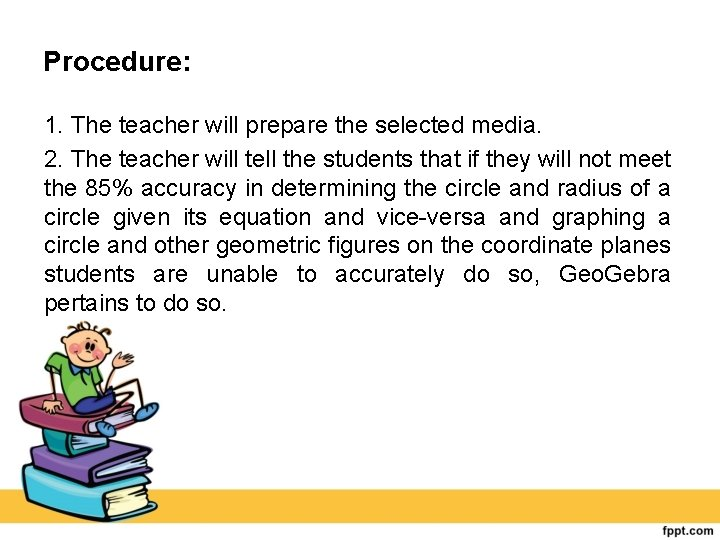Procedure: 1. The teacher will prepare the selected media. 2. The teacher will tell the students that if they will not meet the 85% accuracy in determining the circle and radius of a circle given its equation and vice versa and graphing a circle and other geometric figures on the coordinate planes students are unable to accurately do so, Geo. Gebra pertains to do so.Procedure: 3. The Geo. Gebra app can be used by: a. The teacher will show to input an equation of the circle, shown through LCD screen or projector. b. The students will then follow and try to do it using their phones or laptops, if available. c. After inputting the equation, the app will eventually process and show the graph of the circle’s equation.Procedure: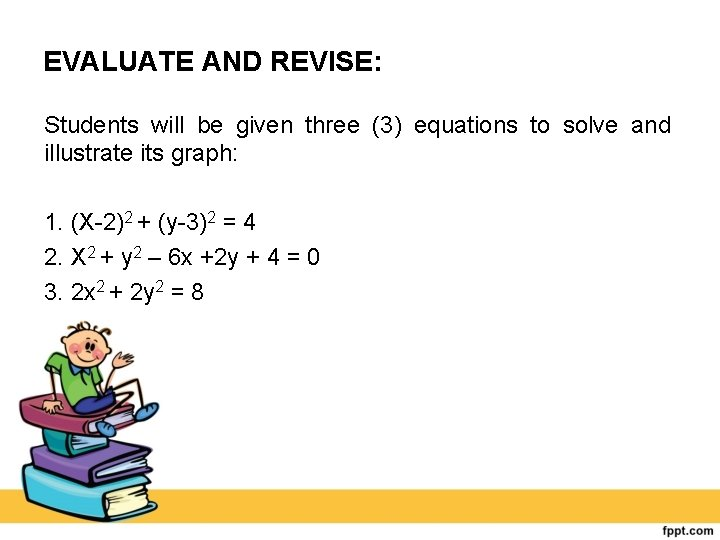EVALUATE AND REVISE: Students will be given three (3) equations to solve and illustrate its graph: 1. (X 2)2 + (y 3)2 = 4 2. X 2 + y 2 – 6 x +2 y + 4 = 0 3. 2 x 2 + 2 y 2 = 8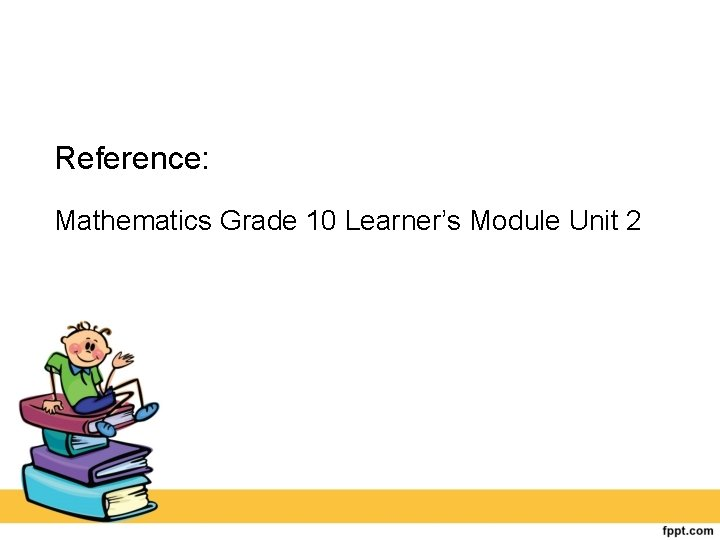Reference: Mathematics Grade 10 Learner’s Module Unit 2SAMR Model: LESSON PLAN IN MATHEMATICS 10I. OBJECTIVES Within the period, students are expected to: Content Standard: a. Demonstrates understanding of key concepts of circle and coordinate geometry.I. OBJECTIVES Performance Standard: a. Is able to formulate and find solutions to challenging situations involving circles and other related terms in different disciplines through appropriate and accurate representations. b. Is able to formulate and solve problems involving geometric figures on the rectangular coordinate plane with perseverance and accuracy.I. OBJECTIVES Learning Competency: a. Illustrates the center radius form of the equation of a circle. b. Determines the circle and radius of a circle given its equation and vice versa. c. Graph a circle and other geometric figures on the coordinate plane.II. SUBJECT MATTER Topic: Reference: Circle Mathematics Grade 10 Learner’s Module Unit 2 Technologies Integrated: Teacher: Will use computers to provide feedback, Projector Students: Will use computers and cellphones to work Multiple Intelligences: Logical/ Mathematical, Verbal/ LinguisticIII. PROCEDURE SAMR: This lesson is beneficial to students because it integrates technology into a Mathematics lesson plan. Students are learning both Mathematic concepts and technology skills both at the same time through the use of various application and technologies. Students may need to use applications such as these throughout the future in college education, so it is nice to start getting them familiar with basic computer function and skills below is how we use SAMR to teach Mathematics lesson.III. PROCEDURE This is an introductory lesson on circles desig ned for grade 10 Mathematics of Catarman National High School. There are 50 grade 10 students composed of 28 girls and 22 boys. This lesson serves as continuation about circles and shows the students how the equation of a circle can be manipulated to change its center and radius.III. PROCEDURE •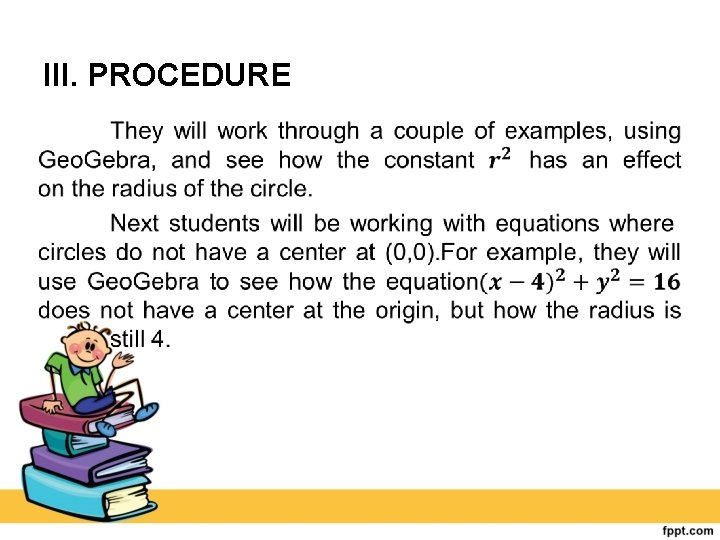III. PROCEDURE •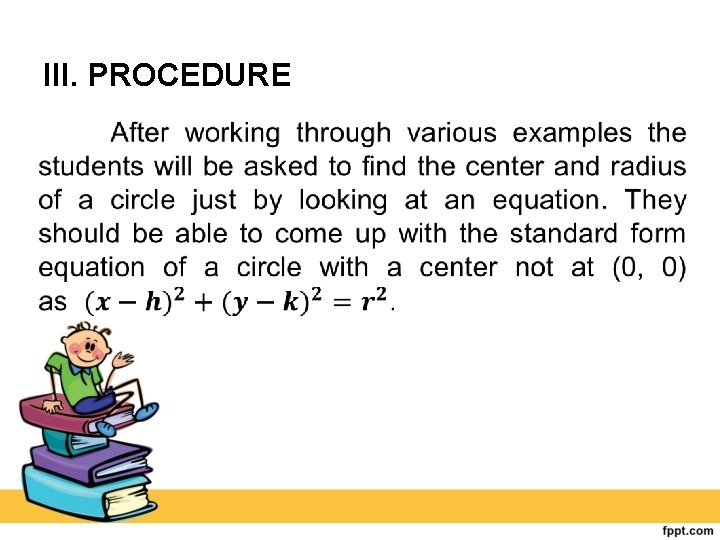III. PROCEDURE •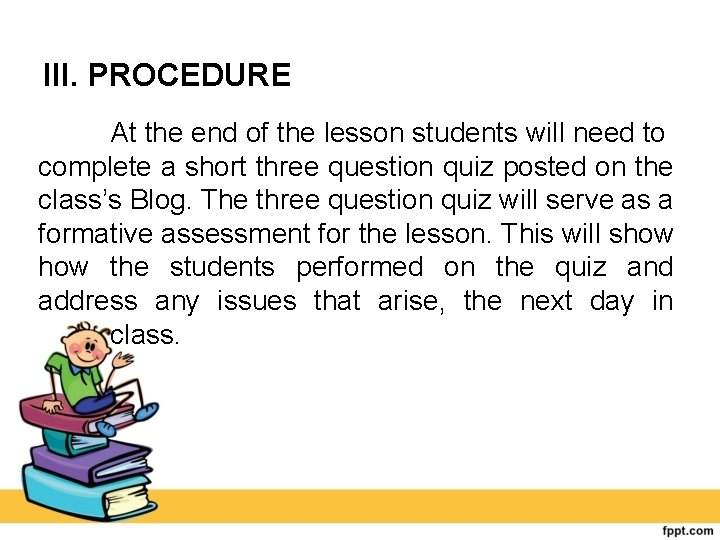III. PROCEDURE At the end of the lesson students will need to complete a short three question quiz posted on the class’s Blog. The three question quiz will serve as a formative assessment for the lesson. This will show the students performed on the quiz and address any issues that arise, the next day in class.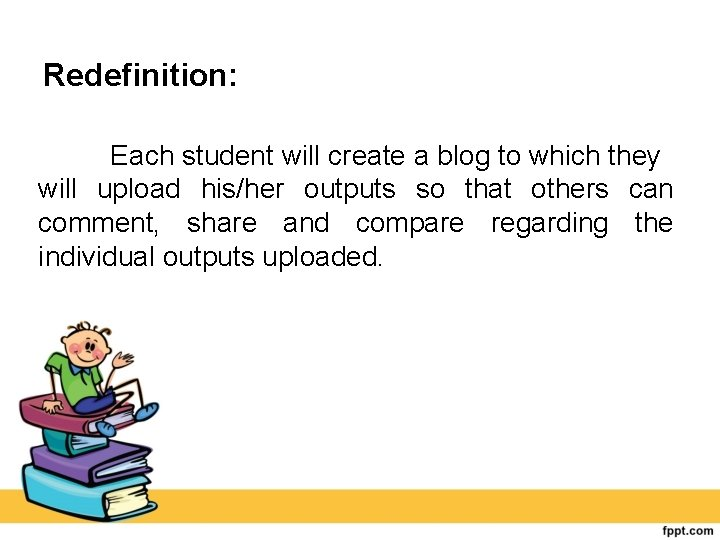Redefinition: Each student will create a blog to which they will upload his/her outputs so that others can comment, share and compare regarding the individual outputs uploaded.Modification: Students screen capture the graph of the circle’s equation they come up on the Geo. Gebra Application (using either laptop or cellphone) and use them to create a screencast (video) about their learning to share with others.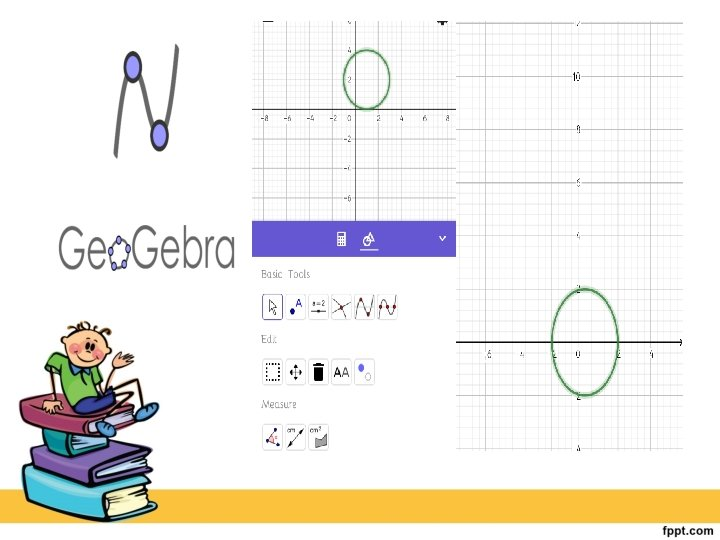Augmentation: Students will use an application called Geo. Gebra Application, which is a technology that mixes geometry with algebra. For this lesson students will be working with concepts from both geometry and algebra. They will be working with circles and seeing how equations can change the graph of a circle. Since this lesson incorporates a combination of geometry and algebra, Geo. Gebra is the ideal technology to use.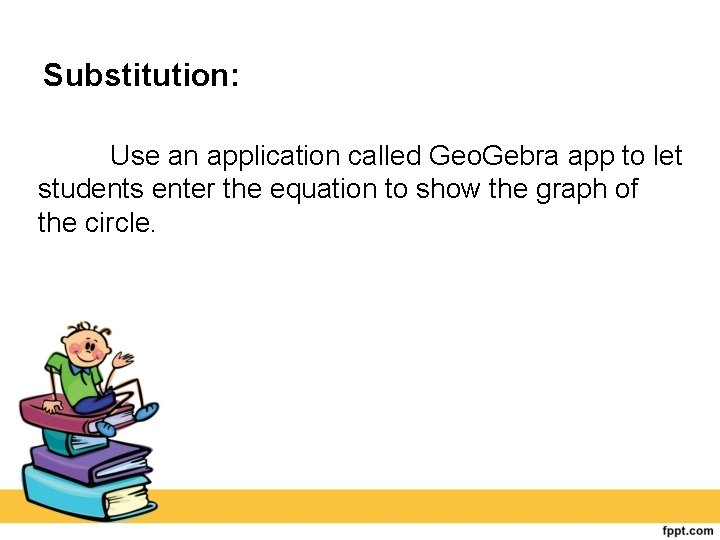Substitution: Use an application called Geo. Gebra app to let students enter the equation to show the graph of the circle.TPACK Model: LESSON PLAN IN MATHEMATICS 10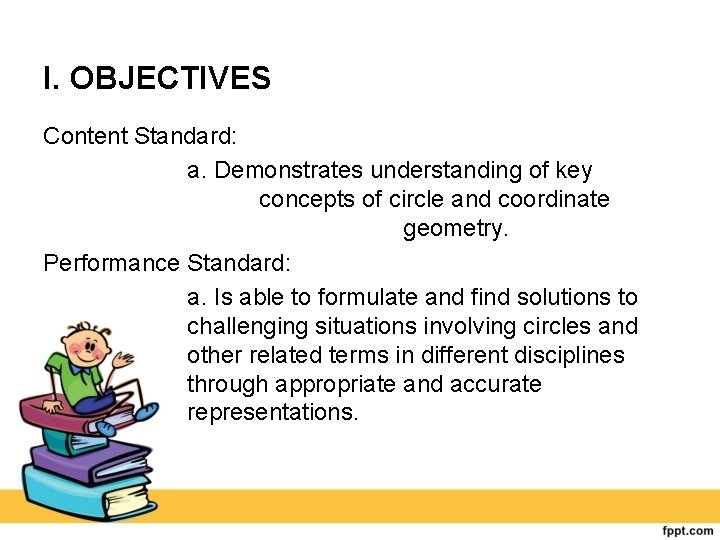I. OBJECTIVES Content Standard: a. Demonstrates understanding of key concepts of circle and coordinate geometry. Performance Standard: a. Is able to formulate and find solutions to challenging situations involving circles and other related terms in different disciplines through appropriate and accurate representations.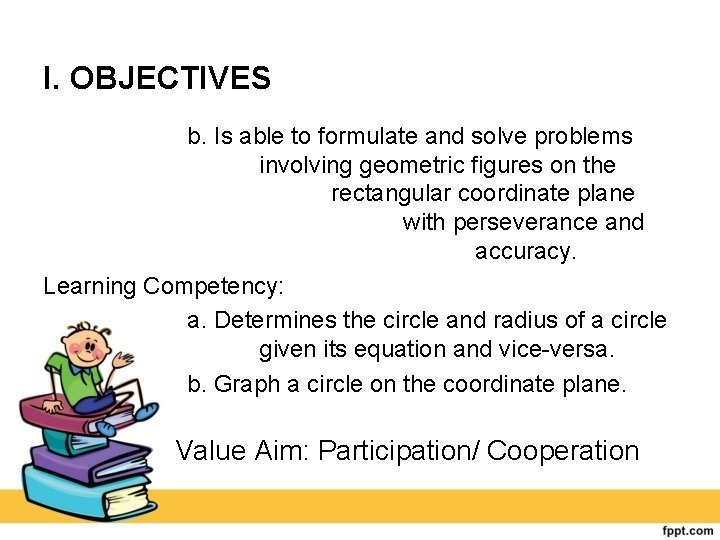I. OBJECTIVES b. Is able to formulate and solve problems involving geometric figures on the rectangular coordinate plane with perseverance and accuracy. Learning Competency: a. Determines the circle and radius of a circle given its equation and vice versa. b. Graph a circle on the coordinate plane. Value Aim: Participation/ Cooperation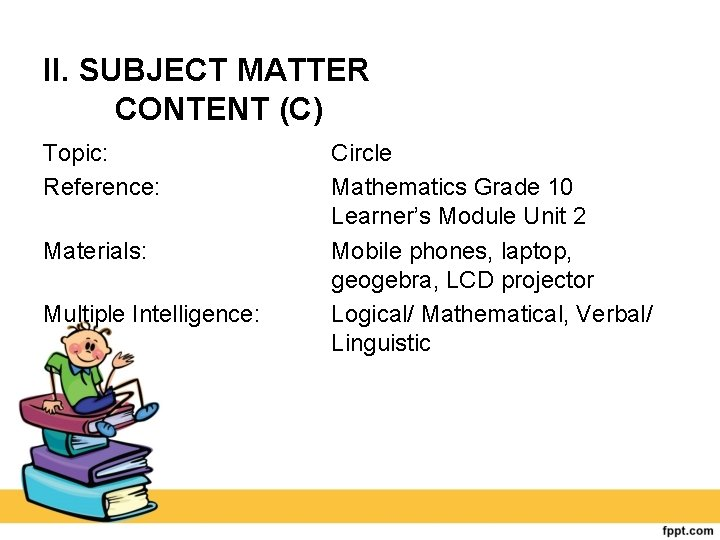II. SUBJECT MATTER CONTENT (C) Topic: Reference: Materials: Multiple Intelligence: Circle Mathematics Grade 10 Learner’s Module Unit 2 Mobile phones, laptop, geogebra, LCD projector Logical/ Mathematical, Verbal/ Linguistic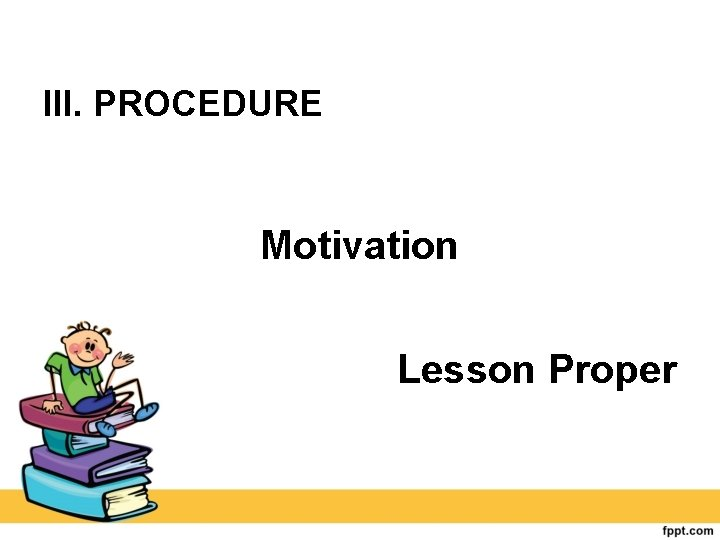III. PROCEDURE Motivation Lesson ProperLesson Content This is an introductory lesson on circles designed for grade 10 Mathematics of Catarman National High School. There are 50 grade 10 students composed of 28 girls and 22 boys. This lesson serves as continuation about circles and shows the students how the equation of a circle can be manipulated to change its center and radius.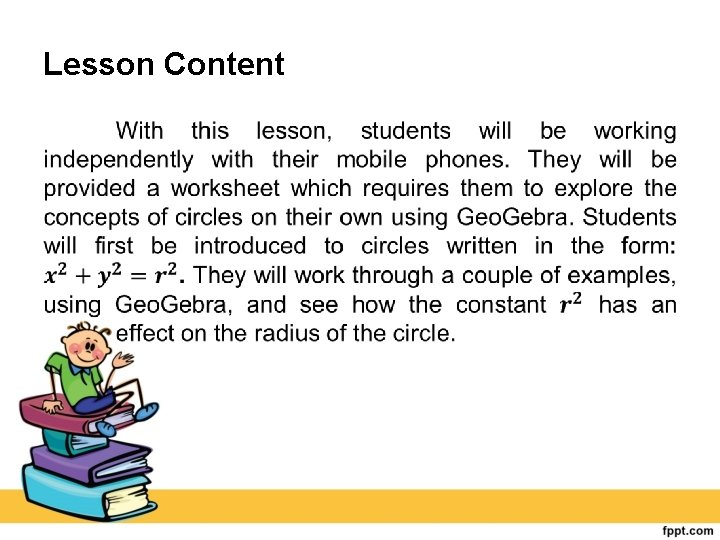Lesson Content •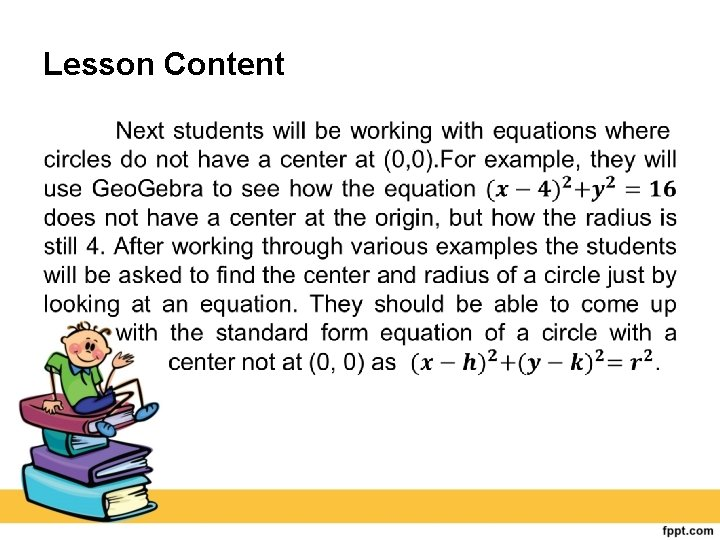Lesson Content •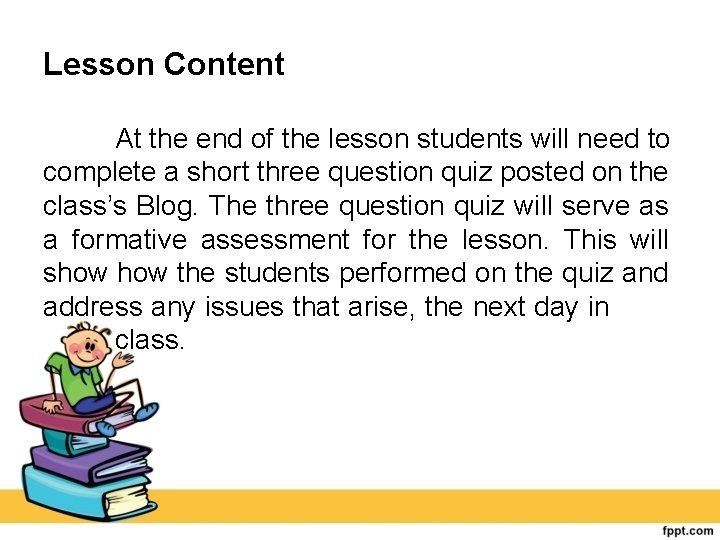Lesson Content At the end of the lesson students will need to complete a short three question quiz posted on the class’s Blog. The three question quiz will serve as a formative assessment for the lesson. This will show the students performed on the quiz and address any issues that arise, the next day in class.PEDAGOGY (P): • Inquiry Based Learning • Experiential Learning TECHNOLOGY (T) • Geo. Gebra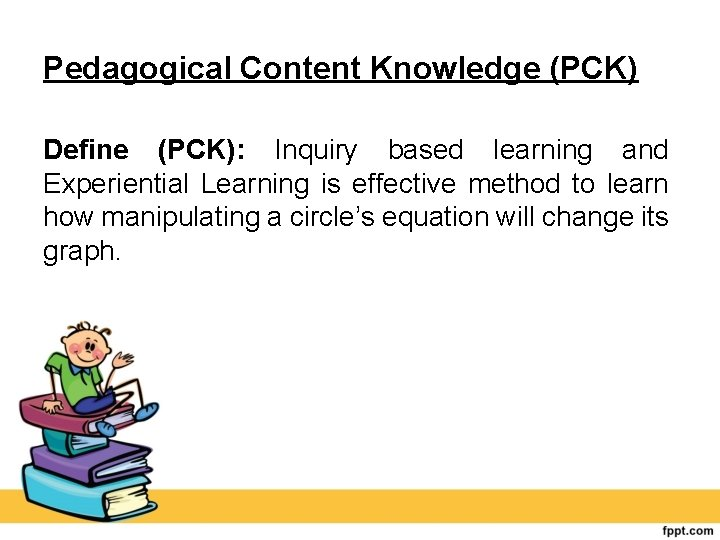Pedagogical Content Knowledge (PCK) Define (PCK): Inquiry based learning and Experiential Learning is effective method to learn how manipulating a circle’s equation will change its graph.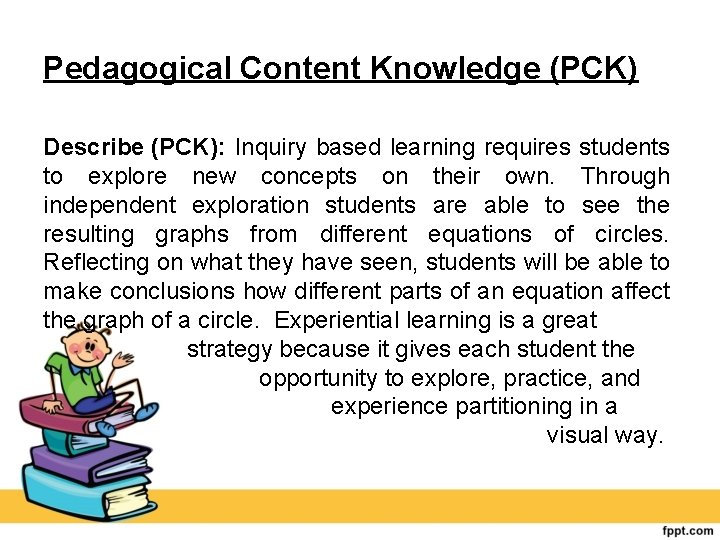Pedagogical Content Knowledge (PCK) Describe (PCK): Inquiry based learning requires students to explore new concepts on their own. Through independent exploration students are able to see the resulting graphs from different equations of circles. Reflecting on what they have seen, students will be able to make conclusions how different parts of an equation affect the graph of a circle. Experiential learning is a great strategy because it gives each student the opportunity to explore, practice, and experience partitioning in a visual way.Pedagogical Content Knowledge (PCK) Support (PCK): Inquiry based learning is a style of learning that focuses around the student and is ideal for a math class. According to Amélie G. Schinck (2011), an inquiry approach to teaching in mathematics leads to a greater retention of concepts by the students. Through inquiry learning students gain deeper procedural knowledge and a better understanding of the concepts.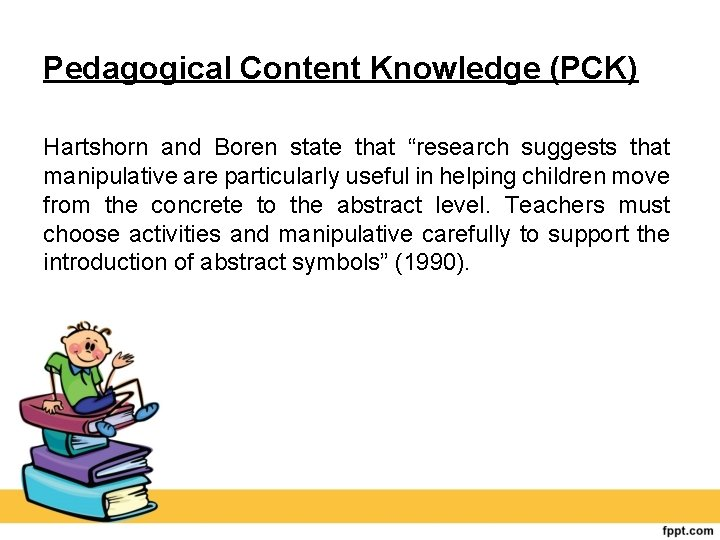Pedagogical Content Knowledge (PCK) Hartshorn and Boren state that “research suggests that manipulative are particularly useful in helping children move from the concrete to the abstract level. Teachers must choose activities and manipulative carefully to support the introduction of abstract symbols” (1990).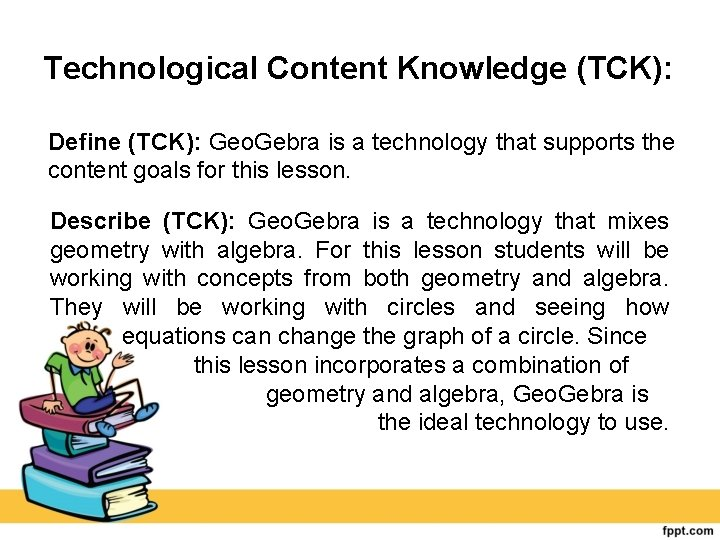Technological Content Knowledge (TCK): Define (TCK): Geo. Gebra is a technology that supports the content goals for this lesson. Describe (TCK): Geo. Gebra is a technology that mixes geometry with algebra. For this lesson students will be working with concepts from both geometry and algebra. They will be working with circles and seeing how equations can change the graph of a circle. Since this lesson incorporates a combination of geometry and algebra, Geo. Gebra is the ideal technology to use.Technological Content Knowledge (TCK): Support (TCK): Geo. Gebra allows students to make connections across a number of different mathematical disciplines. According to Escuder and Furner (2012), “Geo. Gebra is a dynamic geometry system in which you work with points, vectors, segments, lines, and conic sections. Geo. Gebra is also a dynamic algebraic system, where equations and coordinates can be entered directly. ” For this lesson students are being asked to compare equations and graphs of circles. Making Geo. Gebra the ideal technology to use.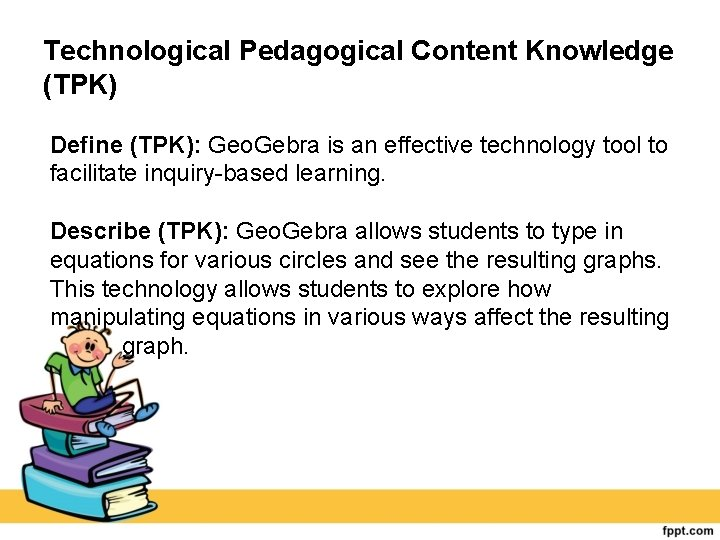Technological Pedagogical Content Knowledge (TPK) Define (TPK): Geo. Gebra is an effective technology tool to facilitate inquiry based learning. Describe (TPK): Geo. Gebra allows students to type in equations for various circles and see the resulting graphs. This technology allows students to explore how manipulating equations in various ways affect the resulting graph.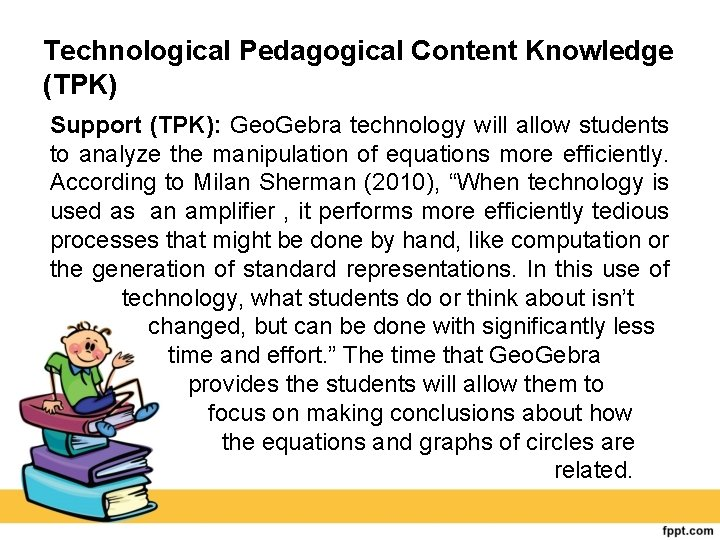Technological Pedagogical Content Knowledge (TPK) Support (TPK): Geo. Gebra technology will allow students to analyze the manipulation of equations more efficiently. According to Milan Sherman (2010), “When technology is used as an amplifier , it performs more efficiently tedious processes that might be done by hand, like computation or the generation of standard representations. In this use of technology, what students do or think about isn’t changed, but can be done with significantly less time and effort. ” The time that Geo. Gebra provides the students will allow them to focus on making conclusions about how the equations and graphs of circles are related.Technological Pedagogical Content Knowledge (TPACK) Support (TPACK): In a study performed by Praveen Shadaan and Leong Kwan Eu (2013), it was found that students who were taught circles using Geo. Gebra had an increase in conceptual understanding compared to those not taught with Geo. Gebra. These observations support the decision to teach the students’ circles through inquiry based learning and experiential learning using Geo. Gebra.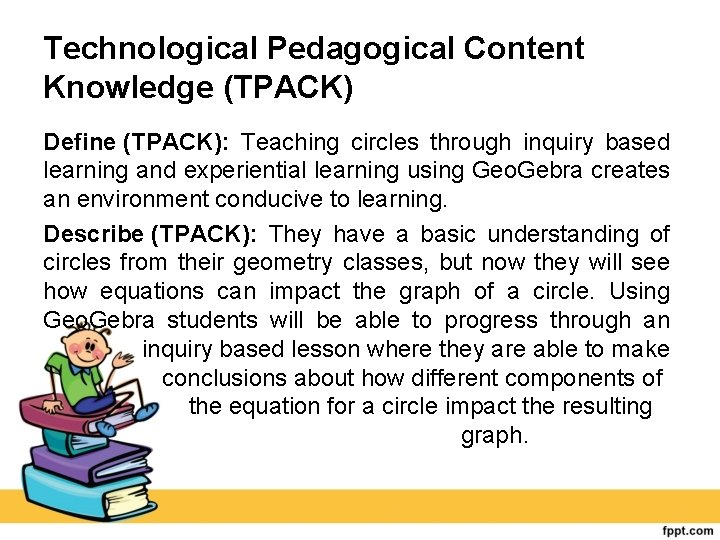Technological Pedagogical Content Knowledge (TPACK) Define (TPACK): Teaching circles through inquiry based learning and experiential learning using Geo. Gebra creates an environment conducive to learning. Describe (TPACK): They have a basic understanding of circles from their geometry classes, but now they will see how equations can impact the graph of a circle. Using Geo. Gebra students will be able to progress through an inquiry based lesson where they are able to make conclusions about how different components of the equation for a circle impact the resulting graph.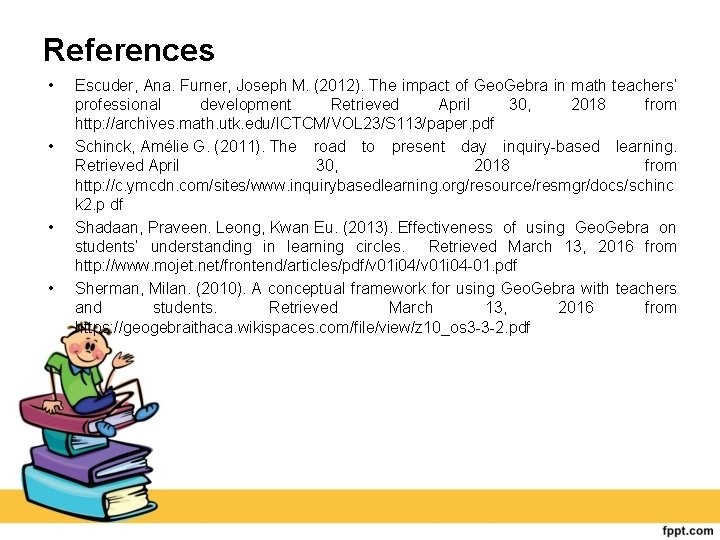References • • Escuder, Ana. Furner, Joseph M. (2012). The impact of Geo. Gebra in math teachers’ professional development Retrieved April 30, 2018 from http: //archives. math. utk. edu/ICTCM/VOL 23/S 113/paper. pdf Schinck, Amélie G. (2011). The road to present day inquiry based learning. Retrieved April 30, 2018 from http: //c. ymcdn. com/sites/www. inquirybasedlearning. org/resource/resmgr/docs/schinc k 2. p df Shadaan, Praveen. Leong, Kwan Eu. (2013). Effectiveness of using Geo. Gebra on students’ understanding in learning circles. Retrieved March 13, 2016 from http: //www. mojet. net/frontend/articles/pdf/v 01 i 04 01. pdf Sherman, Milan. (2010). A conceptual framework for using Geo. Gebra with teachers and students. Retrieved March 13, 2016 from https: //geogebraithaca. wikispaces. com/file/view/z 10_os 3 3 2. pdf